Math resources Geometry Perimeter

Perimeter of a rectangle

# Perimeter of a rectangle

Here you will learn how to find the perimeter of a rectangle, including what the perimeter is, how to calculate it and how to solve perimeter word problems.

Students will first learn how to find perimeter as part of measurement and data in 3 rd grade and 4 th grade.

## What is the perimeter of a rectangle?

The perimeter of a rectangle is the total distance around the outside of the rectangle.

For example,

Perimeter is measured in units. Each side of the square is one unit.

The perimeter of the rectangle is found by counting the units on each side of the rectangle. You can do this by starting at a vertex and counting each unit around the shape until you arrive back at the vertex.

There are 24 units around the outside of the rectangle, so the perimeter is 24 units.

Notice the perimeter can also be found by adding the length of each side.

9 + 3 + 9 + 3 = 24 , so the perimeter is 24 units.

Since the opposite sides of a rectangle are the same, you can also multiply each side by 2 and then add them to calculate the perimeter.

\begin{aligned} & 3 \text { units } \times 2+9 \text { units } \times 2 \\\\ & =6 \text { units }+18 \text { units } \\\\ & =24 \text { units } \end{aligned}

The general perimeter of a rectangle formula is 2 \times \text { length }+2 \times \text { width } .

### What is the perimeter of a rectangle?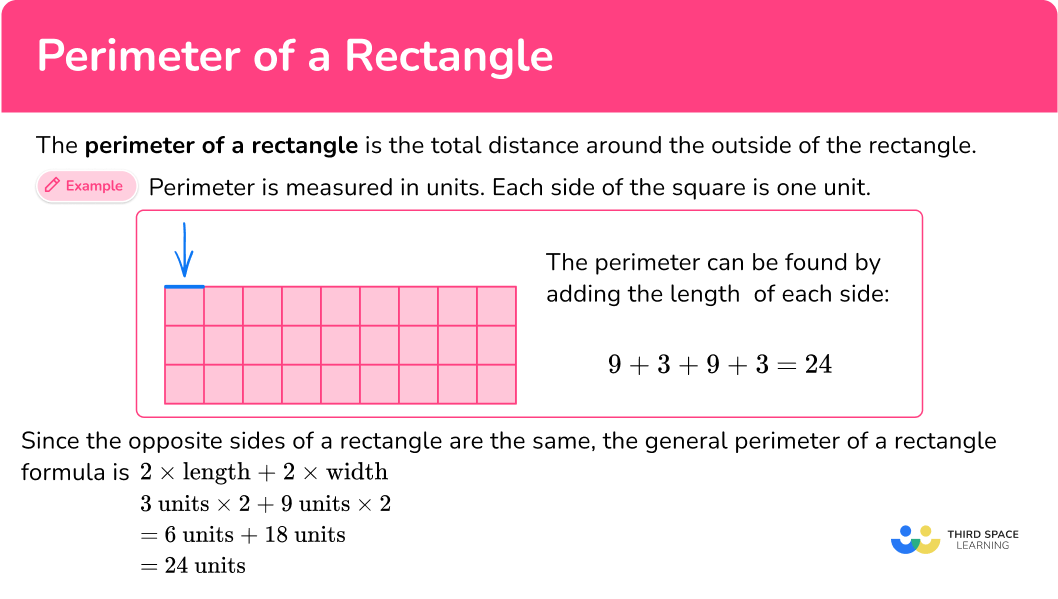## Common Core State Standards

How does this relate to 3 rd grade math and 4 th grade math?

• Grade 3 – Measurement and data (3.MD.D.8)
Solve real world and mathematical problems involving perimeters of polygons, including finding the perimeter given the side lengths, finding an unknown side length, and exhibiting rectangles with the same perimeter and different areas or with the same area and different perimeters.
• Grade 4 – Measurement and data (4.MD.A.3)
Apply the area and perimeter formulas for rectangles in real world and mathematical problems. For example, find the width of a rectangular room given the area of the flooring and the length, by viewing the area formula as a multiplication equation with an unknown factor.

## How to find the perimeter of a rectangle

In order to calculate the perimeter of a rectangle:

1. Add all the side lengths.
2. Write the final answer with the correct units.

## How to find perimeter examples

### Example 1: gridded rectangle

What is the perimeter of the rectangle?

1. Add all the side lengths.

The length of the rectangle is 7 units.

The width of the rectangle is 5 units.

The opposite sides of the rectangle are congruent (equal).

To find the perimeter (the total distance around the rectangle), add all the side lengths:

7 + 5 + 7 + 5 = 24

2Write the final answer with the correct units.

There are no specific units given, so they are just labeled ‘units.’

The perimeter of the rectangle is 24 units.

### Example 2: gridded rectangle

What is the perimeter of this rectangle?

One side, the length of the rectangle, is 13 units.

The other side, the width of the rectangle, is 3 units.

The opposite sides of the rectangle have equal lengths.

To find the perimeter (the total distance around the rectangle), add all the side lengths:

13 + 3 + 13 + 3 = 32

You can also multiply by the \text { length } and the \text { width } by 2 and then add:

\begin{aligned} & 2 \times 13+2 \times 3 \\\\ & =26+6 \\\\ & =32 \end{aligned}

There are no specific units given, so they are just labeled ‘units.’

The perimeter of the rectangle is 32 units.

### Example 3: non-gridded rectangle

What is the perimeter of the rectangle?

The length of the rectangle is 22{~ft} . The width of the rectangle is 3{~ft} . The opposite sides of the rectangle are congruent (equal).

To find the perimeter (the total distance around the square), add all the side lengths:

3 + 22 + 3 + 22 = 50

You can also multiply by the \text { length } and the \text { width } by 2 and then add:

\begin{aligned} & 2 \times 3+2 \times 22 \\\\ & =6+44 \\\\ & =50 \end{aligned}

The side lengths are measured in feet, so the total perimeter is in feet.

The perimeter of the square is 50 feet.

### Example 4: non-gridded rectangle

What is the perimeter of the rectangle?

The length of the rectangle is 34{~mm} . The width of the rectangle is 11{~mm} . The opposite sides of the rectangle are congruent (equal).

To find the perimeter (the total distance around the square), add all the side lengths:

34 + 11 + 34 + 11 = 90

You can also multiply by the \text { length } and the \text { width } by 2 and then add:

\begin{aligned} & 2 \times 34+2 \times 11 \\\\ & =68+22 \\\\ & =90 \end{aligned}

The side lengths are measured in millimeters, so the total perimeter is in millimeters.

The perimeter of the square is 90 {~mm} .

### Example 5: given perimeter – find missing side length

The perimeter of the rectangle is 88 inches. Find the missing side length.

The opposite sides of a rectangle are congruent (equal).

88 inches is the total length around the rectangle so, the perimeter is 21 \, + \, ? + \, 21 + \, ?=88 inches

Since 21 + 21 = 42 , the two missing sides make up the rest of the perimeter.

Subtract to see how much more is needed to get to a total perimeter of 88 :

88 - 42 = 46

So, the combined length of the missing sides is 46 .

You need to solve ? \, + \, ? = 46 . Since the two sides are congruent, each side must be 23 , because 23 + 23 = 46 .

The rectangle is measured in inches.

The missing side length is 23 inches.

### Example 6: perimeter word problem

A rectangular field has a length of 15{~m} and a width of 14{~m} . If the field is outlined in rope, how much rope is needed?

The sides of the field are 15{~m} and 14{~m} , so add them twice to calculate the perimeter.

15+14+15+14=58 \mathrm{~m}

You can also multiply by the \text { length } and the \text { width } by 2 and then add:

\begin{aligned} & 2 \times 15+2 \times 14 \\\\ & =30+28 \\\\ & =58 \end{aligned}

The rectangle is measured in meters, so the perimeter is in meters.

The perimeter of the field is 58{~m} .

### Teaching tips for perimeter of a rectangle

• Instead of just giving students study materials, make sure students have plenty of practice finding the perimeter by tiling rectangles (via physical manipulatives or a digital resource). These opportunities provide the conceptual understanding necessary to understand the perimeter formula.

• Choose worksheets that have a variety of question types – rectangles gridded and non-gridded, solving for the perimeter or a missing side length, word problems with or without visuals.

### Easy mistakes to make

• Thinking the order of adding the sides matters
Since addition is commutative, it doesn’t matter the order in which the sides of the shape are added. The sides equal the same sum as long as all sides are added only once.

• Confusing the formulas or solving strategies for perimeter with area
Since they are often introduced together, in the beginning it is easy to confuse the two vocabulary words and/or concepts. After enough exposure and intentional classroom discourse, students will understand and remember the difference.

• Confusing the units for perimeter with area
If students do not have a complete understanding of why perimeter is units and area is square units, they may mix up when to use each. Providing opportunities such as physically measuring the perimeter of items and working with area on grids, can help build an understanding of the difference.
For example,

• Confusing the units
In order to add the sides together, the width and length of a rectangle need to be in the same units. If the sides are given in different units, convert to one common unit before adding.

### Practice perimeter of a rectangle questions

1) What is the perimeter of the rectangle?20 units24 units12 units4 unitsThe perimeter is the total distance around the outside of the rectangle.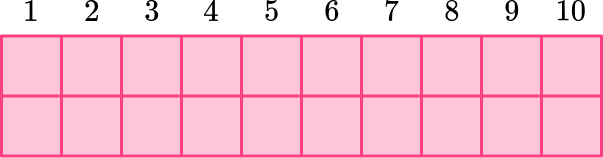The length of the rectangle is 10 units.The width of the rectangle is 2 units.The opposite sides of the rectangle are congruent (equal).

To find the perimeter (the total distance around the rectangle), add all the side lengths:

10 + 2 + 10 + 2 = 24

The perimeter of the rectangle is 24 units.

You can also count each unit of the rectangle to find the perimeter: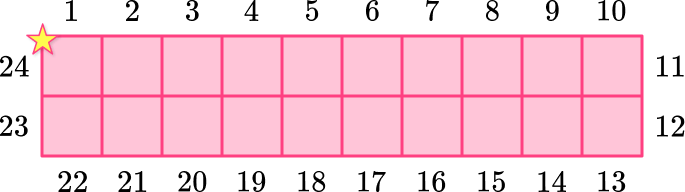2) What is the perimeter of the rectangle?20 units22 units12 units6 unitsThe perimeter is the total distance around the outside of the rectangle.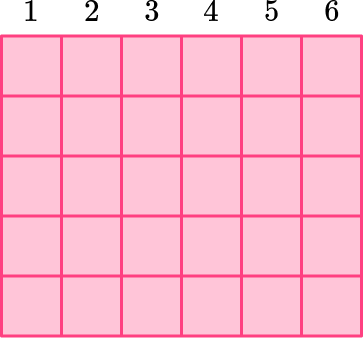The length of the rectangle is 6 units.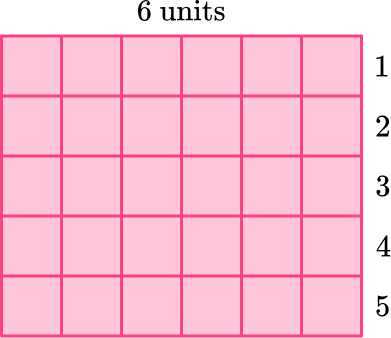The width of the rectangle is 5 units.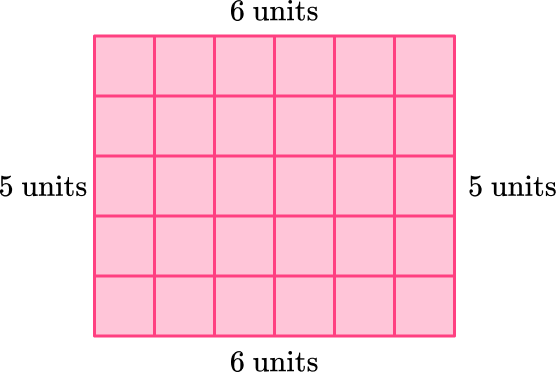The opposite sides of the rectangle are congruent (equal).

To find the perimeter (the total distance around the rectangle), add all the side lengths:

6 + 5 + 6 + 5 = 22

You can also count each unit of the rectangle to find the perimeter: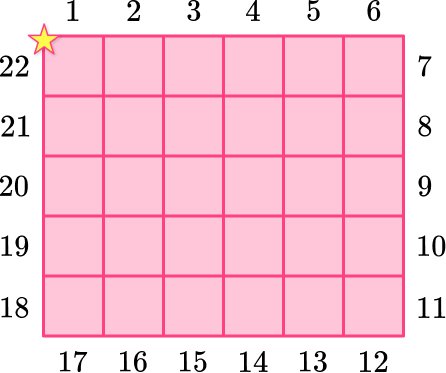The perimeter of the rectangle is 22 units.

3) What is the perimeter of the rectangle?88 square feet132 square feet28 square feet56 feetThe length of the rectangle is 22{~ft} . The width of the rectangle is 6{~ft} . The opposite sides of the rectangle are congruent (equal).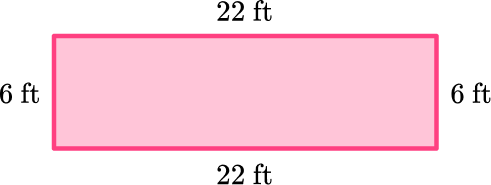To find the perimeter (the total distance around the square), add all the side lengths:

22 + 6 + 22 + 6 = 56

You can also multiply by the \text { length } and the \text { width } by 2 and then add:

\begin{aligned} & 2 \times 22+2 \times 6 \\\\ & =44+12 \\\\ & =56 \end{aligned}

The perimeter of the rectangle is 56 feet.

4) What is the perimeter of the rectangle?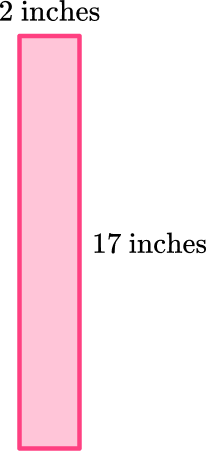15 inches34 square inches19 square inches38 inchesThe length of the rectangle is 2 inches. The width of the rectangle is 17 inches. The opposite sides of the rectangle are congruent (equal).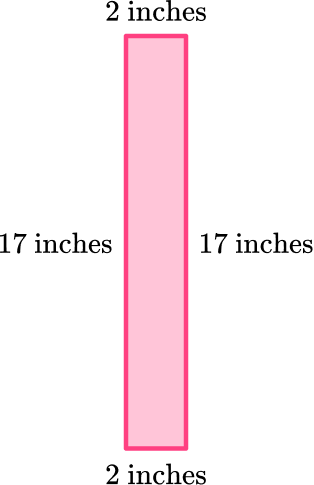To find the perimeter (the total distance around the square), add all the side lengths:

17 + 2 + 17 + 2 = 38

You can also multiply by the \text { length } and the \text { width } by 2 and then add:

\begin{aligned} & 2 \times 17+2 \times 2 \\\\ & =34+4 \\\\ & =38 \end{aligned}

The perimeter of the rectangle is 38 inches.

5) A rectangular garden is 29{~ft} long and 14{~ft} wide. If the garden is outlined with a fence, how much fencing is needed?

43{~ft}86{~ft}74{~ft}91{~ft}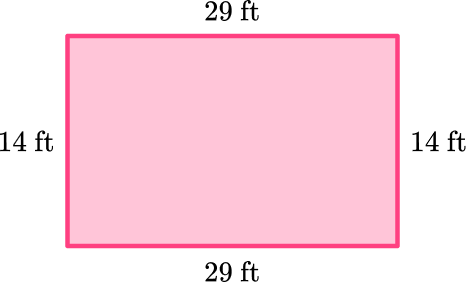The sides of the field are 29{~ft} and 14{~ft} , so add them together twice to calculate the perimeter.

29 + 14 + 29 + 14 = 86 \mathrm{~ft}

You can also multiply by the \text { length } and the \text { width } by 2 and then add:

\begin{aligned} & 2 \times 29+2 \times 14 \\\\ & =58+28 \\\\ & =86 \end{aligned}

The garden needs 86{~ft} of fencing.

6) The perimeter of the rectangle is 100{~m} . Find the missing side length.32{~m}18{~m}36{~m}82{~m}The opposite sides of a rectangle are congruent (equal).

So, the perimeter is \, ? + 18 \, + \, ? + 18 = 100{~m}

Since 18 + 18 = 36 , the two missing sides make up the rest of the perimeter.

Subtract to see how much more is needed to get to a total perimeter of 100 :

100 \, – \, 36 = 64

So, the combined length of the missing sides is 64 .

You need to solve ? \, + \, ? = 64 . Since the two sides are congruent, each side must be 32 , because 32 + 32 = 64 .

The missing side length is 32{~m} .

## Perimeter of a rectangle FAQs

How do you find the perimeter of rectangles?

Since the perimeter of rectangles is the distance around the rectangle, you add the side lengths together to find the total distance.

What is the area of a rectangle?

The area of the rectangle is the number of square units inside the rectangle. The area formula for rectangles is \text { length } \times \text { width } .

How do you find the perimeter of other geometric shapes like a quadrilateral or a parallelogram?

Just like with rectangles, add all the side lengths together to calculate the total distance around the outside of the shape.

## Still stuck?

At Third Space Learning, we specialize in helping teachers and school leaders to provide personalized math support for more of their students through high-quality, online one-on-one math tutoring delivered by subject experts.

Each week, our tutors support thousands of students who are at risk of not meeting their grade-level expectations, and help accelerate their progress and boost their confidence.